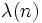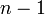# Carmichael number

## Definition

A composite numberis termed an Carmichael number or absolute pseudoprime if it satisfies the following equivalent conditions:

1. The universal exponent (also called the Carmichael function)ofdivides.
2. For any natural numberrelatively prime to,divides.
3.is a Fermat pseudoprime to any base relatively prime to it.
4.is a square-free odd number greater than 1 anddividesfor every prime divisorof.